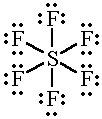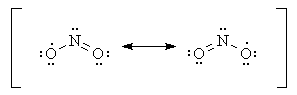# What are the limitations of the octet rule?

Dec 27, 2013

The octet rule states that atoms can combine either by transferring or by sharing their valence electrons to attain an octet of electrons in their valence shell.

#### Explanation:

One limitation of the octet rule is that it cannot be applied to the nonmetals after silicon in the Periodic Table.

These elements can “expand their octet” and have more than eight valence electrons around the central atom.

Examples are${\text{PF}}_{5}$ , ${\text{SF}}_{6}$, and ${\text{H"_2"SO}}_{4}$.

The $\text{P}$ atom in ${\text{PF}}_{5}$ has 10 electrons in its valence shell:The $\text{S}$ atom in ${\text{SF}}_{6}$ has 12 electrons in its valence shell:And so does the $\text{S}$ atom in ${\text{H"_2"SO}}_{4}$:Molecules with an odd number of electrons such as $\text{NO}$ and ${\text{NO}}_{2}$ cannot satisfy the octet rule.

One atom must have an odd number of electrons.

The $\text{N}$ atom in $\text{NO}$ has only 7 electrons in its valence shell.(from file.scirp.org)

In ${\text{NO}}_{2}$, one of the $\text{O}$ atoms has only 7 electrons in its valence shell.In some molecules the central atom cannot possibly have eight valence electrons. For example, ${\text{BeCl}}_{2}$ and ${\text{BCl}}_{3}$ do not obey the octet rule.

$\text{Be}$ has only four electrons in its valence shell.And $\text{B}$ has only six electrons in its valence shell.